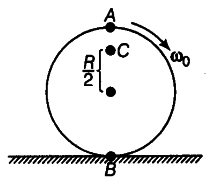# Explain why friction is necessary to make the disc

Explain why friction is necessary to make the disc in figure rolling in the direction indicated.(i) Give the direction of frictional force at Band the sense of frictional torque before perfect rolling begins.
(ii) What is the force of friction after perfect rolling begins?

To roll a disc, one require a linear velocity which can be provided only by a tangential force. As frictional force is the only tangential force in this case, so it is necessary for the rolling of the disc. Initially, friction will be kinetic.
(i) As frictional force at B opposes the angular velocity of B. So, frictional force is in the forward direction, the sense of frictional torque is such as to oppose the angular motion and produce some linear motion, so that the condition of pure rolling (vcω = Rω) should be fulfilled.
(ii) After pure rolling starts there will be no need of friction, so friction force will become zero.